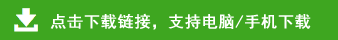# 小学三年级下册数学重点知识检测题

一、 细心计算，我能行（30分）

1、直接计算。（12分）
60×90=  42×49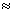7.8+2.1=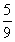+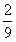=    960÷3=   30×21=
6.3-0.4=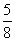-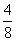=   600÷2÷3=
25×4×5=    54÷9×6=    81+19=
2. 竖式计算（带星的要验算）（18分）
70×45=    528÷9=    9.1-1.8=
★49×72=   ★804÷5=    ★615÷3=

1. 5t=（    ）kg
700平方厘米=（  ）平方分米
8元5角=（   ）元
8000m=（   ）km
16平方米=（    ）平方分米
1年半=（    ）月
2. □65÷5，要使商是三位数，□里最小填（    ）。
3. 钟面上分针的运动方式是（    ）。（填“平移”或“旋转”）
4. 第29届奥运会在中国北京举行，这一年共有（    ）天。
5. 王芳家订一份报纸每个季度要12元，订一年要（   ）元。
6. 填上合适的单位名称。

7. 用分数或小数表示涂色部分。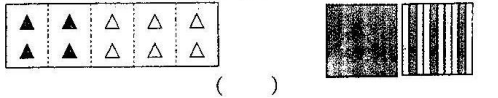8. 这张报纸的三版的面积大约是（    ）平方分米
9. 为残疾人献爱心，三年级有4个班，每班48人，共捐款576元，平均每人捐（    ）元。
10. 玩具汽车124元，大型拼图112元。现在一律按半价出售，小刚带120元买这两样玩具够吗？答：（   ）

1. 李小强离你1千米远，你大声说：“你好！”他（    ）
A一定能听到    B一定听不到      C可能听到也可能听不到